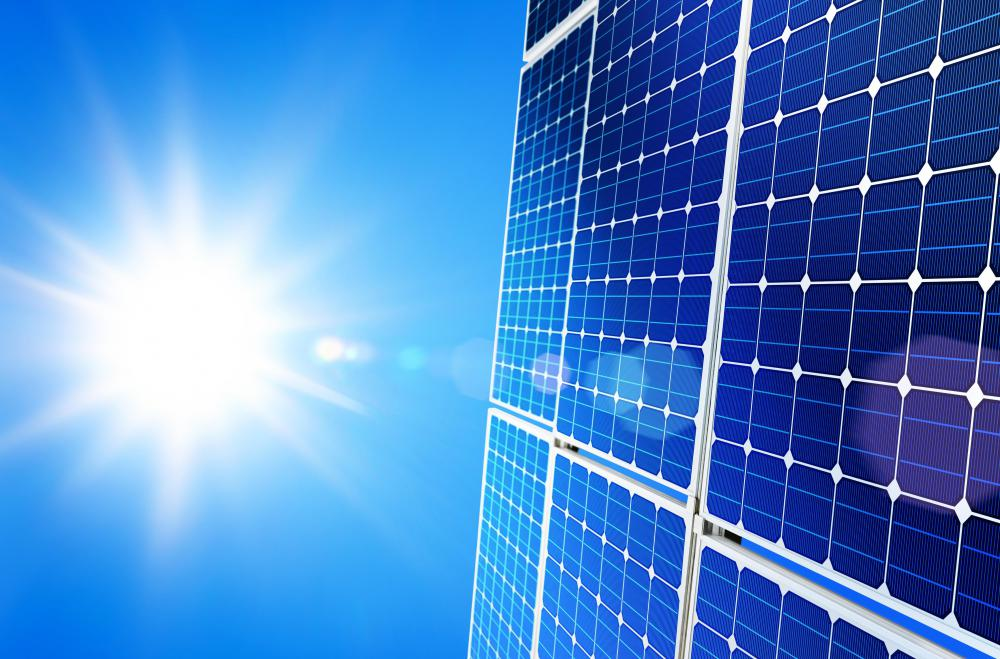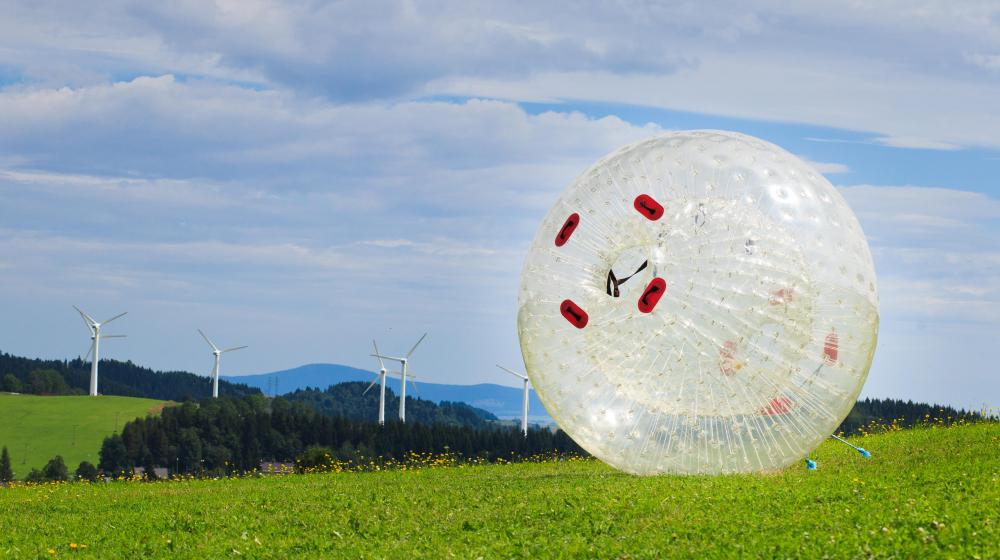# What is the Law of Conservation of Energy?

Vasanth S.

The law of conservation of energy is a principle of physics that states that, in a closed system, energy cannot be created or destroyed. It is expressed in the First Law of Thermodynamics, which states that energy may be transformed into many forms, such as light or heat, but the overall sum of the energies is conserved, or remains constant. Generally, this law is illustrated with a pendulum. The height at which the ball is released at one end of a pendulum will equal the height the ball will reach at the other end. In fact, in a theoretically frictionless environment, the ball will continue to swing back and forth forever.Energy cannot be destroyed, but it can be transformed, as when solar panels convert light and radiation energy from the sun into usable electrical energy.

As a fundamental concept in physics, the law of conservation of energy provides an explanation for how energy is conserved and converted within a system. Generally, one form of energy can be converted into another form of energy. For example, potential energy can be converted to kinetic energy.An example of potential energy is a ball at the top of a hill.

The kinetic energy of a particular object is the energy it posses while in motion. As an expression, kinetic energy equals one-half of the mass of the object, multiplied by the square of the velocity of the object, or KE = 1/2mv2. Kinetic energy consists of three types of energies. Vibrational kinetic energy is the energy due to vibrational motion, and rotational kinetic energy is the energy due to rotational motion. Translational kinetic energy is the energy due to motion of the center of mass from one point to another.

Generally, the potential energy of an object is the energy that is stored while at rest in a force field. Gravity is a force that acts upon an object and gives it potential energy. For example, a ball at the top of a hill has a certain amount of stored energy due to gravity. Other types of potential energy include electric, magnetic, and elastic. An example of elastic potential energy is a stretched spring.

The law of conservation of energy states that the potential energy of a ball on a hill is generally converted to kinetic energy when the ball starts rolling down the hill as a result of gravity. Similarly, the potential energy of a stretched spring becomes kinetic energy when the spring is released. In a pendulum, the law establishes that, when the ball is at its highest point, all the energy is potential energy and there is zero kinetic energy. At the ball's lowest point, all the energy in the ball is kinetic and there is zero potential energy. The total energy of the ball is the sum of the potential energy and kinetic energy.

## You might also Likeanon357739

What is the behind the law that energy is neither created nor destroyed?

anon354977

I really want to know what are its disadvantages? I can't find it anywhere on any website. If you can help, please do.

anon346890

Who established the Law of Conservation of Energy?

anon218447

Babalaas

@ PelesTears- The first law, the law of conservation of enery, has been explained. The second law is the law of entropy. This is basically the law of chaos, stating that energy cannot be returned to the same state, basically implying that entropy increases and a perpetual motion machine is impossible. The third law is the law of absolute zero, stating that absolute zero is unattainable.

There is a zeroth law, which was created afterward to define the other laws. This law describes thermodynamic equilibrium and defines temperature.

PelesTears

What are the other laws of thermodynamics?

Glasshouse

The law of consevation of energy is important in understanding thermodynamics. Any heat gained by one object in a closed system must equal the heat lost by the other object(s) in the system.

I recently did a lab experiment where I used the change in temperature and mass of a piece of hot aluminum dropped into an insulated container of water to determine how much energy was transferred to the container of water.

The law of conservation of energy let me know that the energy transferred into the water is the exact same amount of energy released by the aluminum. the amount of energy released by the aluminum is the equivalent of the mass, change in temperature, and specific heat all multiplied together.